# Intersection Calculations Detail

This document shows, with all the gory details, how the calculations are made for finding the trees that intersect a given GLI light ray. Recall that light rays are defined by an origin point (x, y, z), an azimuth angle, and an altitude angle.

The code begins by calculating a maximum search radius for a given light ray. It takes the tallest possible tree (the largest value in the allometry file for maximum tree height) and then determines the distance at which the tree top just grazes the azimuth angle. (See diagrams below for how that relationship would be worked out.)

The code searches within this maximum radius for trees taller than the z coordinate of the light ray origin. All trees found in this way will be assessed as possible interceptors. (It would be more efficient, of course, to narrow the search based on azimuth direction. However, it's much easier to rip off SORTIE's circle-searching code.)

The code assesses azimuth intersection and altitude intersection separately.

### Determining whether a tree is along the azimuth angle

When determining the azimuth, the code takes a two-dimensional projection of the tree and its neighbor on the forest floor.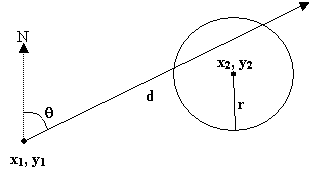In the figure above, the target tree is at (x1, y1) and the neighbor tree is at (x2, y2). The neighbor's canopy radius is r. θ is the azimuth angle of the light ray. To determine whether or not the circle of the neighbor’s canopy intersects the azimuth line, the code solves the circle-and-line system of two equations. The equation for a line is

y = mx + b

where m is the slope of the line and b is the intercept. The slope is given by
m = tan(θ)-1

The equation for a circle is
r2 = (x - h)2 + (y - k)2

where r is the circle radius and the circle center is at point (h, k).

To simplify the problem, the code puts the two trees in their own relative coordinate system, where the target tree is at (0, 0) and the neighbor is at (p, q). Now, in the two equations just given, b = 0, h = p, and k = q. In addition, p = x2 - x1 and q = y2 - y1. So now the equations become

y = mx

and
r2 = (x - p)2 + (y - q)2

Now substitute for y in the second equation, which gives:

r2 = (x - p)2 + (mx - q)2

Simplifying the equation and putting it into quadratic form, we get:
(1 + m2)x2 + (-2p - 2mq)x + p2 + q2 - r2 = 0

An equation in the quadratic form (ax2 + bx + c = 0) has two solutions, which can be found using the quadratic formula: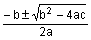For our line-and-circle system of equations,
a = 1 + m2

b = -2p - 2mq

c = p2 + q2 - r2

At this point, though, all the code cares about is whether solutions exist, not what they are (i.e. yes the line and circle intersect, or no they don’t). So the code plugs in m, p, q, and r into the a, b, and c equations to see if b2 - 4ac > 0 and thus has a real square root. If it does, the line and circle intersect; if it doesn’t, they don’t. At this point, we know that a line with slope m intersects the canopy circle, but we are interested in a ray. Trees both along the azimuth and along a line 180 degrees away will have real solutions to the quadratic formula. As a double check, if the quadratic formula has a real solution, the actual azimuth to the tree is taken to make sure it's in the right direction. (It would be easy to assess all the trees directly this way, but when you're assessing a lot of potential trees, the algebraic method is faster.)

### Determining whether a tree is along the altitude angle

If a tree intersects the azimuth angle, it is assessed to see if it also intersects the altitude angle. To determine the altitude, the code again makes the geometry into a two-dimensional problem along the plane of the line projecting from the light ray origin along the azimuth line. In this case the arrangement looks like this: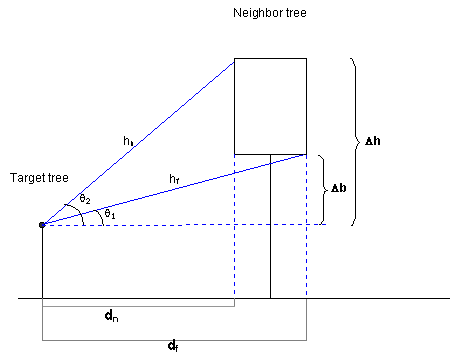Δb is the difference between the height of the light ray origin and the base of the neighbor canopy; Δh is the difference between the height of the light ray origin point and the top of the neighbor canopy; dn is the difference between the target tree trunk and the nearest edge of the neighbor canopy; df is the difference between the target tree trunk and the farthest edge of the neighbor canopy. The code needs to solve for θ1 and θ2 to determine if the light ray's altitude angle is between them.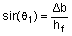and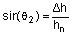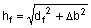and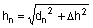To find dn and df, the code finishes solving the line-and-circle system of equations for the two x solutions - call them x3 and x4. These are plugged into the “y = mx” equation to get the y values - call them y3 and y4. The distance from (0, 0) (in our relative coordinate system, the position of the origin of the light ray) to (x3, y3) is dn; from (0, 0) to (x4, y4) is df. So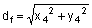and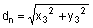After solving for θ1 and θ2, if the light ray's altitude angle is between them, the tree intersects the ray.

### Special case: Overhanging tree canopy

In some cases, a tree's canopy extends out above the light ray's origin point. In this case, assessing whether the ray intersects is done a little differently.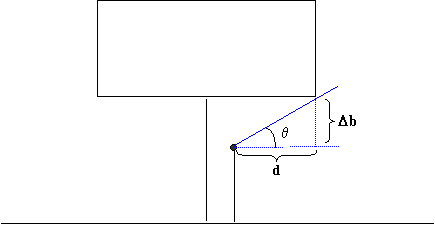In this situation, the canopy always intersects the azimuth angle of the light ray and extends from the altitude angle of the bottom of the canopy (θ) to the zenith. So it only needs to be determined whether the altitude angle of the light ray is above or below the altitude angle of the bottom of the canopy in the plane along the azimuth angle (θ).

The code starts by solving the circle-and-line system of equations as described above to find the two points at which a line of the correct slope intersects the tree canopy. Again, though, we are working with a ray, not a line; one of the points is along the azimuth heading and the other is 180 degrees away. The code determines which solution is along the azimuth heading and throws the other away.

Call the intersection point of the azimuth line and the tree canopy (X3, Y3). Then: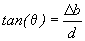and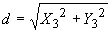The code solves for θ. If it is less than or equal to the altitude angle of the light ray, the tree intersects the ray.